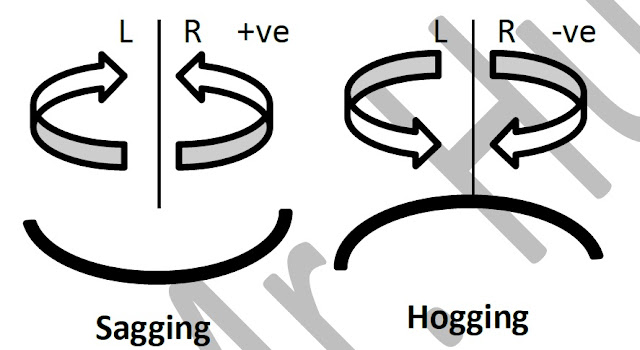# ieCivil

Engineering the Digital Civilization

## Friday, 17 November 2017

Structural Analysis is about Plotting SFD & BMD to derive an idea of the forces the members are subjected to

Many times while studying or practicing the fundamentals of structural design I come across this problem, what were the conventions that are arbitrarily used for plotting an SFD or BMD? and this becomes a serious problem. So as a solution I decided to write a post so that I can bookmark all the basic fundamentals that are put into action while performing this academic task.

For analyzing any structure, first the SFD that is the Shear force diagram is plotted.

### Here are the conventions that are being used:Sign Conventions For Shear Force

### This is how a typical SFD would appear likeShear Force Diagram Generally, all questions in the academic curriculum require you to plot a BMD and elastic deformation curve for the given set of loading, so here's how the sign conventions for BMD are determined.

### Here are the conventions that are being used:Sign Conventions for Bending Moment
If the resultant of the forces makes the element sag, then it is considered as positive else its' considered negative.Either sagging or hogging both induce stresses in the member which could be better explained from the image below:

Finally once you've determined the BMD conventions and calculated the BMD, your final solution for the question would be something as follows:

This was all the text, I assume a video could explain the concepts to a better extent

### Check out the video below to learn how to plot the SFD & BMD

1.2.